Fractional Calculus and Variational Mechanics

Introduction

First of all, let's define fractional calculus, since it is a not-so-well-known term, and it will be the basis of this report. Fractional calculus is the theory of derivatives and integrals of arbitrary non-integer (even complex) order, and started in 1695 when Leibniz presented his analysis of the derivative of order ½. Then it developed mainly as a theoretical aspect of Mathematics, being considered by some of the great names in Mathematics such as Euler, Lagrange, and Fourier, among many others.

Well, what's this about non-integer-order derivatives and integrals?, and more importantly, is it just a theoretical curiosity or does it have practical applications? Recently, fractional calculus has experienced a revival of interest and has been used in very diverse topics such as fractal theory , viscoelasticy , electrodynamics [3,4], optics [5,6], and thermodynamics , to name a few.

The purpose of this report is to review the application of fractional calculus to variational mechanics, more precisely, the ability to account for non-conservative systems with a fractional Lagrangian formulation [8-10]. Amazingly, Lagrangian (or Hamiltonian) mechanics only describe conservative systems, and there have been some approaches at describing non-conservative systems that will also be presented. Riewe [8,9] proposed an elegant (and general) way to incorporate non-conservative systems to the field of variational mechanics using fractional calculus. Since we are not aiming for a description of fractional calculus, we shouldn't dwell on it for too long; however it it useful to have a quick review of the basic concepts behind it, because we will use them later on. If you are already familiar with fractional calculus, you can continue to the next section (although you should make sure to at least look at the notation we will be using throughout the rest of the report).

Previous Attempts to Describe Non-Conservative Systems

Since variational mechanics is a very elegant and powerful formalism when it comes to solving a wide variety of problems in classical mechanics, there have been many approaches meant to include non-conservative systems in such formalism. One of the best known methods is that presented by Rayleigh, introducing a new functional R (which is now called Rayleigh's dissipation function [11,12]), and rewrite the Euler-Lagrange equation in the following way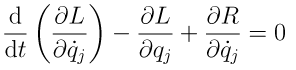where L is the system's (traditional) Lagrangian, and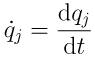.

This provides us with a way to treat dissipative systems, incorporating forces that are functions of the velocity; however, most of the beauty and power of Lagrangian (Hamiltonian) mechanics is that the analyzed system is characterized with only one scalar functional (the Lagrangian L, or the Hamiltonian H). If we would use Rayleigh's dissipation function, then it would take two scalar functionals to completely define the problem. This is the principal objection to this approach.

As pointed out in [8,9,13,14], several non-conservative problems have been solved using the variational formalism. This is done constructing a Lagrangian that leads to differential equations that are equivalent to the ones obtained with Newton's laws. However, such Lagrangians lead to Hamiltonians and momentum equations that are physically misleading. As an example, we could look at the damped linear oscillator described by Ray  but also in [12,13,16], and reviewed by Riewe [8,9]. We would like to obtain the following equation of motion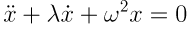where λ is the damping coefficient. If we use the Lagrangian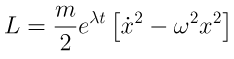or, in general we could use a Lagrangian of the form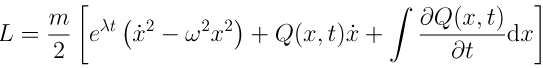where Q = Q(x,t) is arbitrary but continuously differentiable. For this case, we will use Q=0. This leads to the Euler-Lagrange equation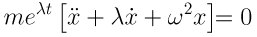Hamiltonian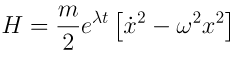and generalized momentum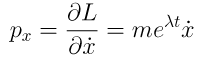We can see that the obtained Euler-Lagrange equation is equivalent to the one we wanted, if we ignore the factor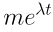,

but the Hamiltonian and conjugate momentum are not quite what we wanted. In fact, it has been pointed out by Ray  that this Lagrangian actually describes an oscillator with varying mass, not a dissipative oscillator. He then proposes two systems (without dissipation) that are described (with physical meaningful momentum and Hamiltonian), by such a Lagrangian:

1. A simple pendulum undergoing small amplitude oscillations which is accreting mass at a rate.
2. An L-C circuit with increasing inductance and decreasing capacitance according to the given exponential law.

Yet another method is that of incorporating an auxiliary coordinate that describes the reverse-time system with negative friction. However, the Hamiltonian leads to additional solutions that must be suppressed, and (again) the momenta are not physically meaningful [8,9].

One can also include the microscopic description of the dissipation into the Lagrangian, but this gets really complicated really fast, and is not a viable way to include dissipation into variational mechanics.

Using Fractional Calculus in Lagrangian Mechanics

So far, the presented approaches are not completely satisfactory when it comes to dealing with non-conservative variational mechanics. Now we describe a method proposed by Riewe [8,9] that, using fractional calculus, offers a powerful way of describing non-conservative systems. Our Lagrangian will be a functional of the generalized coordinates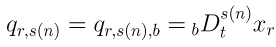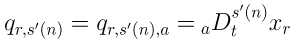where s(n) is any non-negative number (just derivatives, not integrals), and s(0)=0, so that qr,s(0) denotes the coordinate xr (we have labeled coordinates as usual in Physics and Mathematics literature, e.g. x1=x, x2=y, x3=z). In this notation, s(n) is a list of the orders of the derivatives used in the problem, and n is the index used for labeling them.

Since summations over the r index will always be over all values, the convention of summing over repeated indices is used; however, summations over n are not always over all valued, thus all summations over n will be indicated explicitly.

The limits a and b come from the fact that we are looking for an extremum for the action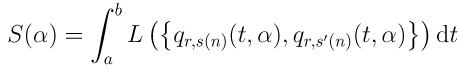where α is the variational parameter . Next, the generalized momenta are defined,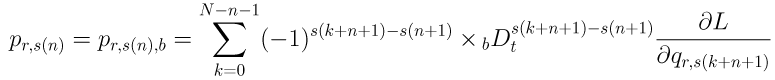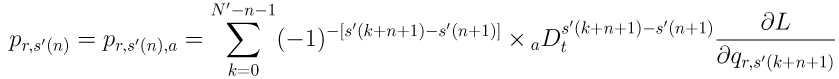and the generalized Euler-Lagrange equation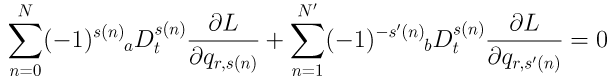Then, the Hamiltonian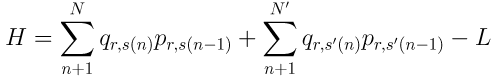and Hamilton's equations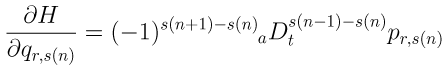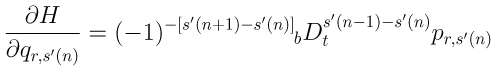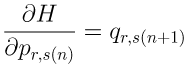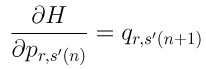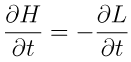For integer-order derivatives, it is shown in detail on  that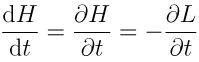so, if the Lagrangian does not depend explicitly on time, then the Hamiltonian is a constant of the motion. However, when we have fractional derivatives in our Lagrangian, this is not generally true and the Hamiltonian is not a constant of the motion, allowing us to account for non-conservative systems in variational mechanics.

This report is not meant to discuss the (extensive) mathematical detail involved in the derivation of the above equations, but to review a new method for describing non-conservative systems in variational mechanics, and illustrate its power through an example, which is worked out in the following section.

A Worked Example: Linear Friction

Now we put the described machinery to work on a simple problem, the same one we have been comparing all along: the linear damped oscillator. We begin with the regular Lagrangian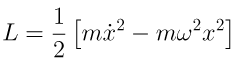and add the term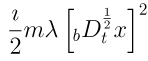in order to get the full fractional Lagrangian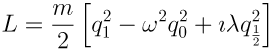where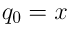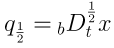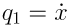and the Euler-Lagrange equation, which is what we wanted!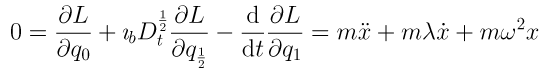then get the generalized momenta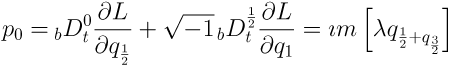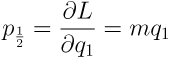so we can write down the Hamiltonian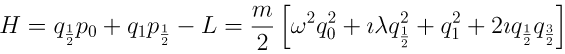and Hamilton's equations just follow from this and give us the same Euler-Lagrange equation (as expected), and identities or definitions, which do not bring new insight and are very easy to work out. Other examples are presented by Rabei et al. .

A (Very Vast) Introduction to Fractional Calculus

There are several definitions of fractional derivatives and integrals, but the most general is the one of Riemann-Liouville. The notation used is not unique, but here we will use the so-called Davis notation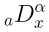to denote the operator for the α-th order fractional integral operator with respect to x based on the lower limit of integration a.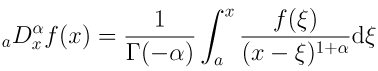for α < 0 and x > a. In the case of fractional derivation (α > 0), to avoid the problems of Γ(-α) when α = 1,2,3,... we use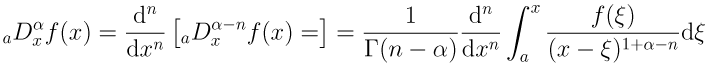where n is the smallest integer exceeding α, this is (n-1) ≤ α < n. It is easy to verify that this reduces to our previous knowledge on integral calculus for integer α. From now on we will refer to derivatives and integrals as differintegrals.

Probably the first thing that we notice about fractional differintegrals is that they depend on the value of the lower limit of integration, which means that the are nonlocal.

Now, let's look into some of the most fundamental properties of the fractional differintegral operator:

• Fractional differintegrals are distributive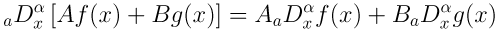• The fractional derivative of a constant is not always zero. However, the fractional differintegral of zero is always zero.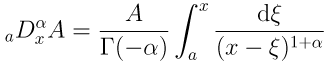• Commutation rule for fractional integrals. Consider two positive real numbers p > 0, q > 0. If f(x) is continuous for x > a, then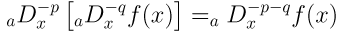• Commutation of fractional derivatives. For two positive numbers 0 ≤ m-1 ≤ p < m, and 0 ≤ n-1 ≤ q < n, the derivative operators (in general) do not commute, as shown by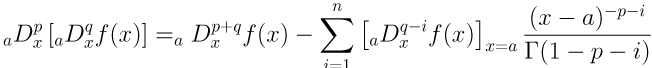• Combination of fractional derivatives and integrals. If f(x) is continuous for x > a, then for both cases p < q and p > q,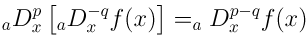and the special case where p = q shows that the fractional derivative operator is left inverse to the fractional integral operator of the same order.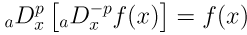• Commutation of fractional derivatives and integrals. As with conventional integer-order differentiation and integration, fractional differentiation and integration do not commute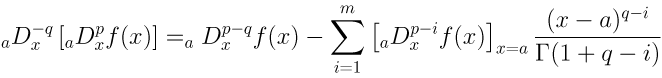Also, fractional derivatives can be approximated using finite differences, and there are several definitions for this (different expressions that converge to the same limit). We will only consider forward and backward differences. For forward differences,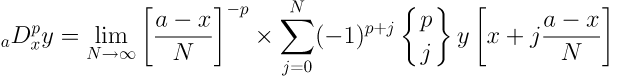and for backward differences,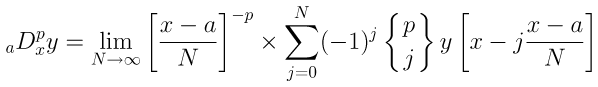where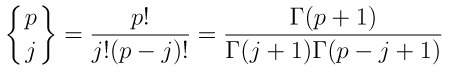is the binomial coefficient.

© 2007 R. J. Noriega-Manez. The author grants permission to copy, distribute and display this work in unaltered form, with attribution to the author, for noncommercial purposes only. All other rights, including commercial rights, are reserved to the author.

References

 A. Carpinteri and F. Mainardi, Fractals and Fractional Calculus in Continuum Mechanics, (Springer, 1997).

 V. V. Novikov and K. V. Voitsekhovskii, "Viscoelastic Properties of Fractal Media," J. App. Mech. Tech. Phys. 41, 149 (2000).

 N. Engheta, "On the Role of Fractional Calculus in Electromagnetic Theory," IEEE Ant. Prop. Mag. 39, 35 (1997).

 T. Kang-Bo et al., "Electrodynamic Analysis of Dissipative Electromagnetic Materials Based on Fractional Derivative," Chinese Phys. Lett. 24, 847 (2007).

 J. C. Gutierrez-Vega, "Fractionalization of Optical Beams: I. Planar Analysis," Opt. Lett. 32, 1521 (2007).

 J. C. Gutierrez-Vega, "Fractionalization of Optical Beams: II. Elegant Laguerre-Gauss Modes," Opt. Express 15, 6300 (2007).

 A. Gaies and A. El-Akrmi, "Fractional Variational Principle in Macroscopic Picture," Physica Scripta 70, 7 (2004).

 F. Riewe, "Nonconservative Lagrangian and Hamiltonian Mechanics," Phys. Rev. E 53, 1890 (1996).

 F. Riewe, "Mechanics with Fractional Derivatives," Phys. Rev. E 55, 3581 (1997).

 D. Baleanu, "Fractional Hamilton Formalism within Caputo's Derivative," Czech. J. Phys. 56, 1087 (2006).

 R. J. Duffin, "Pseudo-Hamiltonian Mechanics," Arch. Rat. Mech. Anal. 9, 309 (1962).

 H. Goldstein, Classical mechanics (Addison-Wesley, 1951).

 D. D. Vujanovic and S. E. Jones, Variational Methods in Nonconservative Phenomena (Academic Press, 1989).

 Y. Huang and C. Lin, "A Systematic Method to Determine the Lagrangian Directly from the Equations of Motion," Am. J. Phys. 70, 741 (2002).

 J. R. Ray, "Lagrangians and Systems They Describe - How Not to Treat Dissipation in Quantum Mechanics," Am. J. Phys. 47, 626 (1979).

 S. T. Thornton and J. B. Marion, Classical Dynamics of Particles and Systems (Thomson Brooks Cole, 2004).

 E. M. Rabei et al., "On Hamiltonian Formulation for Non-Conservative Systems," Turk. J. Phys. 28, 213 (2004).